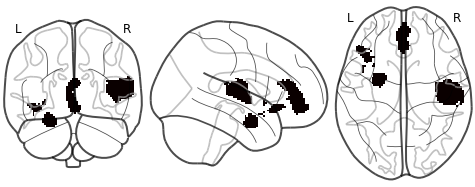I have a statistical mask image containing ones and zeros. I would like to calculate how much the 1-voxels spread over the total space of the image. Note that I do not want to calculate the global volume taken by the voxels (this would be just a substracting the volume taken by the mask voxels substracted from the global volume and then checking how much space they consume) but how strongly they are distributed throughout the brain. So for example, in one image the same amount of voxels could be packed together in one spot whereas in the other image the same number of voxels is spread over a larger area. I am looking for a way to measure this spread.

I already had the idea to just calculate the average inter-voxel-distance. But this algorithm doesn’t work when the number of 1-voxels becomes to big, because you end up with a extreme big number of to-be-calculated distances.

Does anyone has a good alternative idea how this could be done?I’m not aware of any canonical approach for that. Conceptually, this amounts to computing the entropy of the spatial distribution, which would consist of fitting a relatively generic distribution (e.g. Gassian mixture) to the voxel coordinates, and then reporting the entropy of the distribution.
HTH,
Bertrand

1 Like